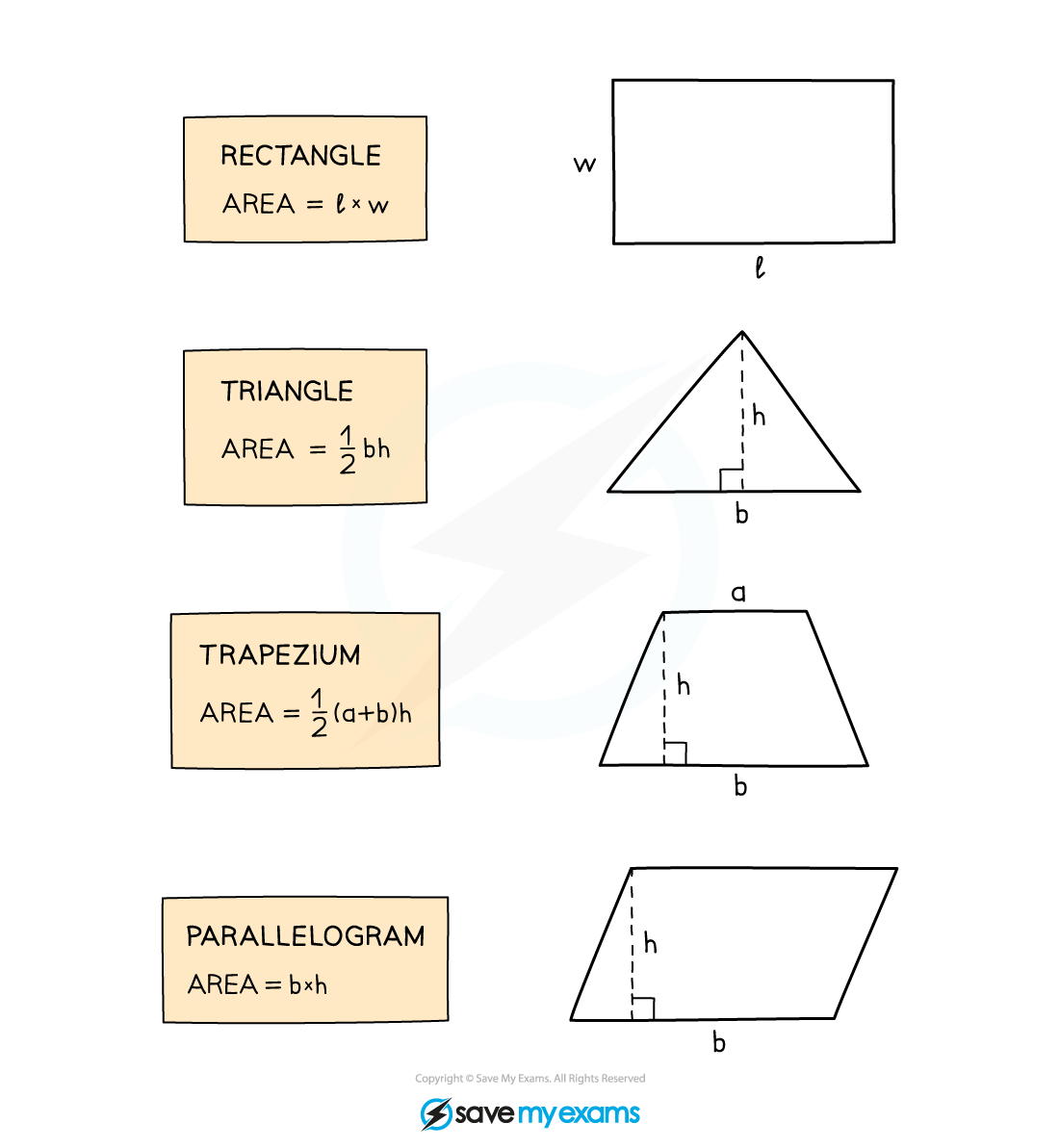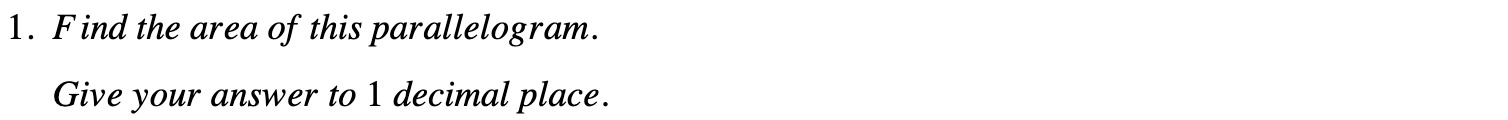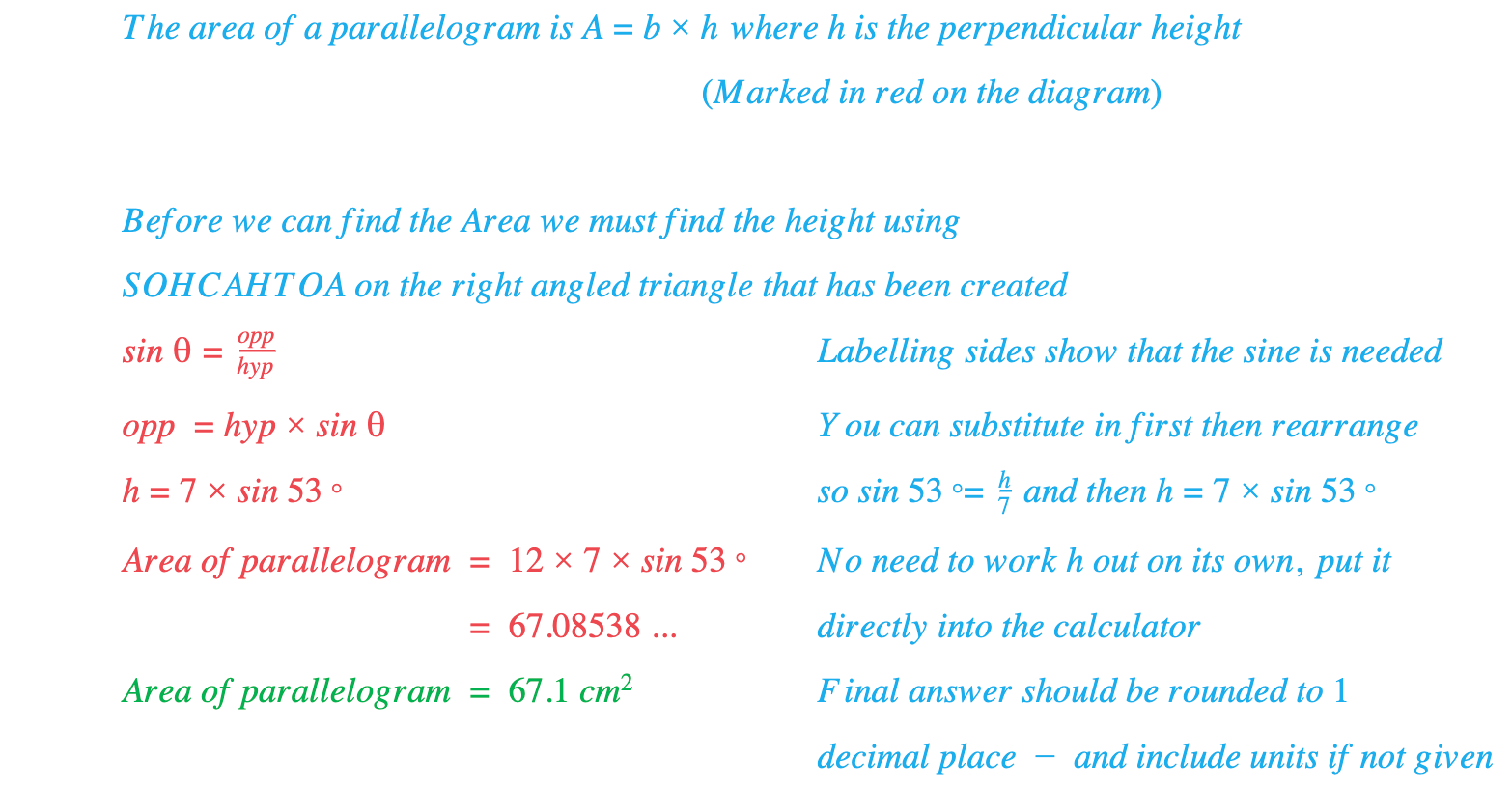# Edexcel IGCSE Maths 复习笔记 4.11.1 Area - Formulae

Edexcel IGCSE Maths 复习笔记 4.11.1 Area - Formulae

#### What is area and why do we need to calculate it?

• Area is the amount of space taken up by a two-dimensional shape
• Volume deals with three-dimensional shapes and space
• Some of the uses of area are a little more obvious than some areas of maths
• Examples include working out the area of a floor if laying or purchasing a new carpet or the amount of land needed if designing a sports field

#### Area – using formulae

• There are some basic formulae you should know and be comfortable using
• Be aware that some area formulae use distances that aren’t necessarily one of the sides of the shape
• Make sure you know what the different letters in each formula are referring to

• These formulae are essential – anything more complicated will be given in the exam:#### Exam Tip

You may have to do some work to find the lengths first – using Pythagoras Theorem, Trigonometry (SOHCAHTOA) etc. so make sure you look out for that!

#### Worked Example#### Worked Example